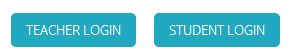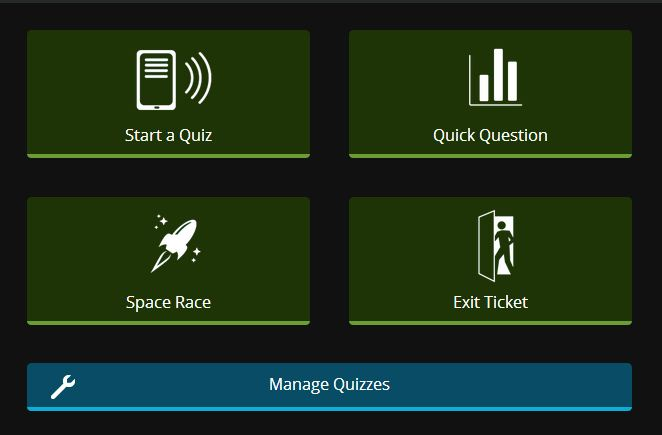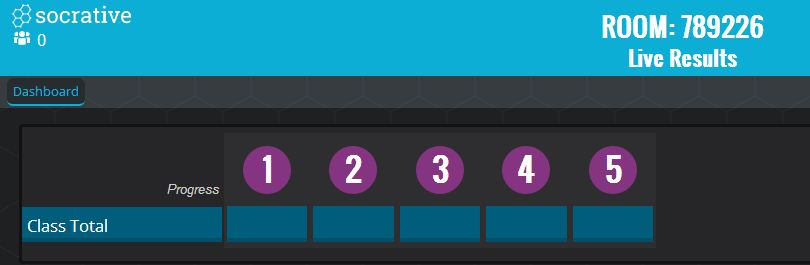# 07 Gravitation

## Escape Velocity

Using the GeoGebra app above, I intend to demonstrate the relationship between total energy, kinetic energy and gravitational potential energy in a rocket trying to escape a planet’s gravitational field.

By changing the total energy of the rocket, you will increase the initial kinetic energy, thus allowing it to fly further from the surface of the planet. The furthest point to which the rocket can fly can be observed by moving the slider for “distance”. You will notice that the furthest point is where kinetic energy would have depleted.

Gravitational potential energy of an object is taken as zero at an infinite distance away from the source of the gravitational field. This means gravitational potential energy anywhere else takes on a negative value of $\dfrac{-GMm}{r}$. Therefore, the total energy of the object may be negative, even after taking into account its positive kinetic energy as total energy = kinetic energy + gravitational potential energy.

The minimum total energy needed for the rocket to leave the planet’s gravitational field is zero, as that will mean that the minimum initial kinetic energy will be equal to the increase in gravitational potential energy needed, according to the equation $\Delta U = 0 – (-\dfrac{GMm}{R_P})$, where $R_P$ is the radius of the planet.

Since $\dfrac{1}{2}mv^2 = \dfrac{GMm}{R_P}$, escape velocity, $v = \sqrt{\dfrac{2GM}{R_P}}$.

## How to Understand the Image of the Black Hole

Here’s a good explanation by Veritasium on why the image of the black hole looks the way it does.

## Simulation for Gravitational Field Strength and Potential

This simulation allows students to observe the variation of gravitational field strength and potential between two masses. Field strength is shown as vectors whereas potential is shown as scalar values on a plot. The resultant field strength and potential are shown in red.

## Getting Tides Right

I was reading up on tides this morning as I have to relieve a colleague’s IP2 class on forces, when I stumbled upon this video. It explains tides in a way that differs from most textbooks that clarified for me why the oceans bulge at both ends along the Moon-Earth line at the 2’30” mark onwards.

## Using Socrative for Pop Quizzes

Since almost every student owns a smartphone in SIngapore now, it has become possible for us to gather instant feedback or conduct an on-the-spot formative assessment to identify common misconceptions during class time.

A simple true-and-false quiz conducted during my recent lesson on Gravitation, after the lecture series was completed, showed that students are confused about the different treatment between vectors (force and field strength) and scalars (potential and potential energy).

1. If you were sitting half as far from your nearest classmate, the gravitational force of attraction between the two of you would be four times as strong. (81.0% correct)
2. At the midpoint of the distance from the center of the earth to the center of the moon, the gravitational field strength is zero. (90.5% correct)
3. At the midpoint of the distance between two identical masses, the gravitational potential is zero. (23.8% correct)
4. Work done by gravitational force in bringing an object from infinity to the surface of a planet is negative. (38.1% correct)
5. Satellites in a geostationary orbit must move directly above the equator. (52.4% correct)

This quiz was effective in helping students realise that potential cannot cancel out.

I also took the opportunity to explain to the class why the definition of gravitational potential (as well as potential energy) was based on the work done by an external force, rather than work done by gravitational force.

The tool that I used was Socrative and if anyone would like to use my quiz, you can follow the steps below:

1. Create a teacher account (not a student account) at socrative.com and log in.2. Click on Manage Quizzes.3. Key in the SOC number 12289667

Since you have imported the quiz, you have effectively duplicated the quiz for yourself and are at liberty to edit the questions or even modify the quiz altogether as it does not affect mine.

To conduct the quiz, all you need to do is to go back to the dashboard and click on “Start a Quiz”, selecting the quiz you have saved. Follow the rest of the instructions to select the type of quiz you want (whether at students’ own pace or at a pace determined by yourself).

Once you have started the quiz, get your students to log in at m.socrative.com on their smartphone browsers and key in your Room Number (shown at the top of the screen). You will be able to see the number of students logging in at the top left corner and the attempts of your students in the main area.If you have any questions regarding the use of Socrative in teaching, feel free to leave a comment below and I’ll try to answer it. Have fun!